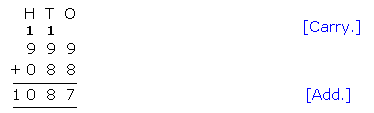## Definition Of Arithmetic

Arithmetic is a branch of mathematics, which usually deals with addition, subtraction, multiplication, and division of numbers.

Arithmetic is considered as the most elementary branch of mathematics.
Arithmetic also includes fractions and percents as they are related to division.
Arithmetic also includes exponents as they are related to multiplication.

### Examples of Arithmetic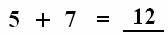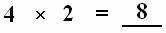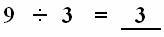### Solved Example on Arithmetic

#### Ques: Add: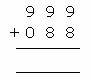##### Choices:

A. 1,807
B. 1,087
C. 1,067
D. 1,077
Step 1: